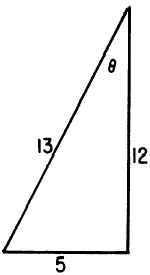Tables of decimal valuesCustom SearchTABLES Tables of decimal values for the trigonometric ratios may be constructed in a variety of ways. Some give the angles in degrees, minutes, and seconds; others in degrees and tenths of a degree. The latter method is more compact and is the method used for appendix II. The "headings" at the bottom of each page in appendix II provide a convenient reference showing the minute equivalents for the decimal fractions of a degree. For example, 12’ (12 minutes) is the equivalent of 0.2°. Finding the Function Value The trigonometric ratios are sometimes called FUNCTIONS, because the value of the ratio depends upon (is a function of) the angle size. Finding the function value in appendix II is easily accomplished. For example, the sine 35° is found by looking in the "sin" row opposite the large number 35, which is located in the extreme left-hand column. Since our angle in this example is exactly 35°, we look for the decimal value of -the sine in the column with the 0.0° heading. This column contains decimal values for functions of the angle plus 0.0°; in our example, 35° plus 0.0°, or simply 35.0°. Thus we find that the sine of 35.0° is 0.5736. By the same reasoning, the sine of 42.7° is 0.6782, and the tangent of 32.3° is 0.6322. A typical problem in trigonometry is to find the value of an unknown side in a right triangle when only one side and one acute angle are known. EXAMPLE: In triangle ABC (fig. 19-8), find the length of AC if AB is 13 units long and angle CAB is 34.7°.Figure 19-8.-Using the trigonometric ratios to evaluate the sides. SOLUTION:The angles of a triangle are frequently stated in degrees and minutes, rather than degrees and tenths. For example, in the foregoing problem, the angle might have been stated as 34° 42’. When the stated number of minutes is an exact multiple of 6 minutes, the minute entries at the bottom of each page in appendix II may be used. Finding the Angle Problems are frequently encountered in which two sides are known, in a right triangle, but neither of the acute angles is known. For example, by applying the Pythagorean Theorem we can verify that the triangle in figure 19-9 isFigure 19-9.-Using trigonometric ratios to evaluate angles. a right triangle, The only information given, concerning angle q, is the ratio of sides in the triangle. The size of q is calculated as follows:Assuming that the sides and angles in figure 19-9 are in approximately the correct proportions, we estimate that angle q is about 20°. The table entries for the tangent in the vicinity of 20 are slightly too small, since we need a number near 0.4167. However, the tangent of 22° 36’ is 0.4163 and the tangent of 22° 42’ is 0.4183. Therefore, q is. between 22° 36’ and 22° 42 ‘.Integrated Publishing, Inc. - A (SDVOSB) Service Disabled Veteran Owned Small Business